Courses

# Test: Quantitative Techniques

## 20 Questions MCQ Test Mock Test Series for CLAT 2022 | Test: Quantitative Techniques

Description
This mock test of Test: Quantitative Techniques for CLAT helps you for every CLAT entrance exam. This contains 20 Multiple Choice Questions for CLAT Test: Quantitative Techniques (mcq) to study with solutions a complete question bank. The solved questions answers in this Test: Quantitative Techniques quiz give you a good mix of easy questions and tough questions. CLAT students definitely take this Test: Quantitative Techniques exercise for a better result in the exam. You can find other Test: Quantitative Techniques extra questions, long questions & short questions for CLAT on EduRev as well by searching above.
QUESTION: 1

### How much above the cost price should a man mark his goods, so that, after allowing a discount of 10% for cash payment, he may still make a profit of 8%?

Solution: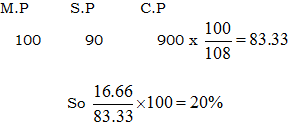QUESTION: 2

### Two numbers are respectively 20% and 50% more than a third number. These two numbers are in the ratio:

Solution: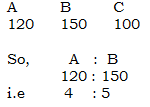QUESTION: 3

### If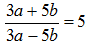, then a : b is equal to:

Solution:

Go via options (d) option satisfies it.

QUESTION: 4

The ratio of the present ages of puneet and Appu is 2 : 3. After 3 years the ratio of their ages will be 3 : 4. The present age of Puneet is:

Solution:

Ratio (1) = 3 yrs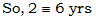QUESTION: 5

Annual incomes of A and B are in the ratio 4 : 3 and their annual expenses are in the ratio 3 : 2. If each of them saves Rs. 600 at the end of the year, what is the annual income of A?

Solution: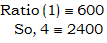QUESTION: 6

The average of marks in Mathematics for 5 students was found to be 50. Later, it was discovered that in the case of one student the marks 48 were misread as 84. The correct average is:

Solution:

Out – 84
In  - 48
Diff = 36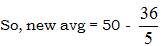= 42.8

QUESTION: 7

If the cost price of 10 articles is equal to the selling price of 7 articles, then the gain or loss per cent is:

Solution:

C.P (10) = S.P (7)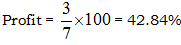QUESTION: 8

Two bicycles were sold for Rs. 3990 each, gaining 5% on one and losing 5% on other. The gain or loss per cent on the whole transaction is:

Solution: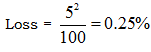QUESTION: 9

Two trains are running with speeds 30 km/hr and 58 km/hr in the same direction. A man in the slower train passes the faster train in 18 seconds. The length (in metres) of the faster train is:

Solution: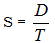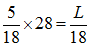So, L = 140 m

QUESTION: 10

In a school 40% of the students play football and 50% play cricket. If 18% of the students neither play football nor cricket, the percentage of the students playing both is:

Solution:

F + C = 90%
But, 100% - 18% = 82%
So, Diff = 90% - 82% = 8%

QUESTION: 11

If the side of a square is increased by 25%, then its area is increased by:

Solution: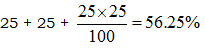QUESTION: 12

A student has to secure 40% marks to pass. He gets 90 marks and fails by 10 marks. Maximum marks are:

Solution: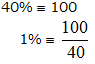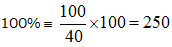QUESTION: 13

If A’s height is 10% more than B’s height, by how much per cent less is B’s height than that of A?

Solution: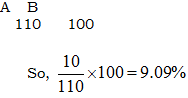QUESTION: 14

A reduction of 20% in the price of sugar enables a purchaser to obtain 3 kg more for Rs. 120. The original price (per kg) of sugar is:

Solution:

Reduction = 20%
Increase in quantity = 1/4
So 1/4 = 3 kg
So, original price = 120/12 = 10

QUESTION: 15

If the difference between the simple and compound interests on a sum of money for 2 years at 4% per annum is Rs. 80, the sum is:

Solution: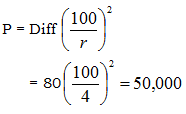QUESTION: 16

A number, when divided by 119, leaves a remainder of 19. If it is divided by 17, it will leave a remainder of:

Solution: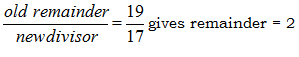QUESTION: 17

The L.C.M. of two numbers is 20 times their H.C.F. The sum of H.C.F. and L.C.M. is 2520. If one of the numbers is 480, the other number is:

Solution:

L x H = a x b
20 H x H = 480 x b
L + H = 2520
So, b = 600

QUESTION: 18

The number, whose square is equal to the difference between the squares of 975 and 585, is:

Solution:

x2 = (975)2 – (585)2
x2 = 608400
So, x = 780

QUESTION: 19

A tap can fill a cistern in 8 hours and another tap can empty it in 16 hours. If both the taps are open, the time (in hours) taken to fill the tank will be:

Solution: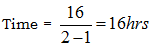QUESTION: 20

Out of 10 teachers of a school, one teachers retires and in his place a new teacher of age 25 years joins. As a result of it, the average age of the teachers is reduced by 3 years. The age of the retired teacher is

Solution:

Age = 25 + 3(10)
= 55 years